# Mixing rulesThis article is a 'stub' page, it has no, or next to no, content. It is here at the moment to help form part of the structure of SklogWiki. If you add sufficient material to this article then please remove the {{Stub-general}} template from this page.

## van der Waals mixing rules

The van der Waals equation of state can be written as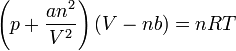$\left(p + \frac{an^2}{V^2}\right)\left(V-nb\right) = nRT$

For mixtures one replaces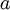$a$ and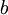$b$ with expressions that depend on the composition: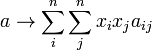$a \rightarrow \sum_i^n \sum_j^n x_i x_j a_{ij}$

and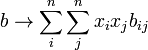$b \rightarrow \sum_i^n \sum_j^n x_i x_j b_{ij}$

where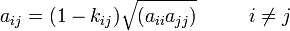$a_{ij} = (1-k_{ij}) \sqrt{(a_{ii} a_{jj})} ~~~~~~~~ i\neq j$

and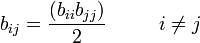$b_{ij} = \frac{(b_{ii} b_{jj})}{2} ~~~~~~~~ i\neq j$

where$k_{ij}$ is obtained from a fit.